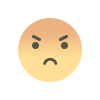# AAO Exam-CT 7: Quant (DI - Bar Graph)

## AAO Exam-CT 7- Quant (DI - Bar Graph)

0  320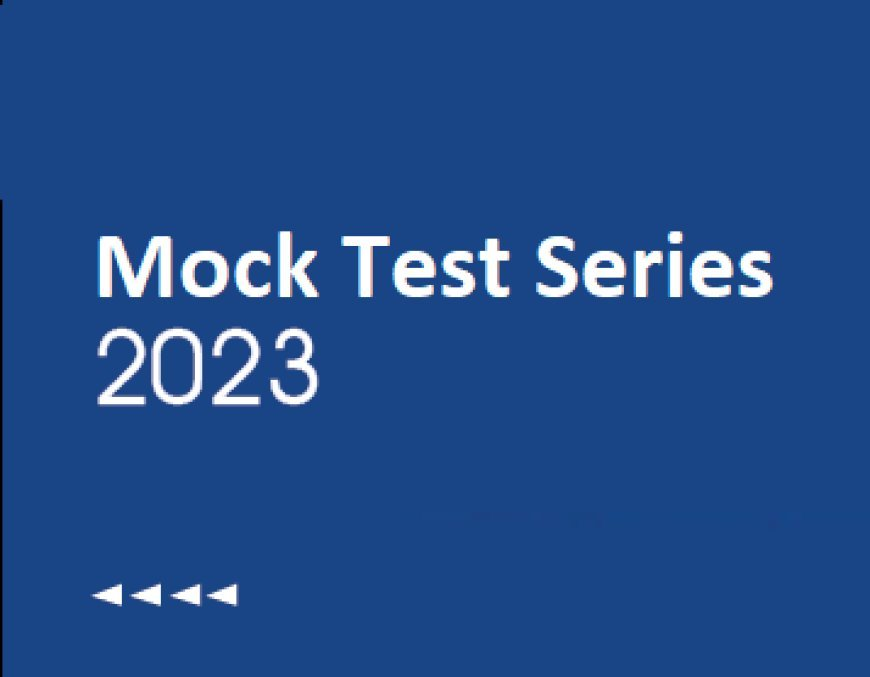1. Direction Read the following graph carefully and answer the following questions∶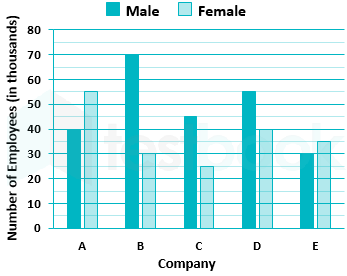Question:
Find the difference between the number of male employees and female employees of five companies.
• 50000
• 61000
• 51000
• 59000
• 55000

Total number of female employees of companies (in thousand) = 55 + 30 + 25 + 40 + 35

⇒ 185000

Total number of male employees of companies (in thousand) = (40 + 70 + 45 + 55 + 30)

⇒ 240000

Required difference = 240000 - 185000 ⇒ 55000

2. Direction Read the following graph carefully and answer the following questions∶Question:
Find the male employees of company D are approximately what percent of total male employees of five companies?
• 29%
• 28%
• 30%
• 23%
• 26%

Calculation

Total number of male employees of companies = 40 + 70 + 45 + 55 + 30

⇒ 240 thousands

Male employees of a company D = 55 thousand

Required percentage = (55 / 240) × 100

⇒ 22.91% ≈ 23% ∴ The required percentage is 23%

3. Direction Read the following graph carefully and answer the following questions∶Question:
Find the average number of employees in the five companies.
• 88000
• 85000
• 81000
• 80000
• 87000

Calculation

Total number of female employees of companies = (55 + 30 + 25 + 40 + 35)

⇒ 185 thousands

Total number of male employees of companies = (40 + 70 + 45 + 55 + 30)

⇒ 240 thousands

Total employees in five companies = 185 + 240

⇒ 425 thousands

Required average = 425 / 5

⇒ 85 thousands

The required average is 85 thousands

4. Direction: The given bar graph shows the number of pens sold by two shops A & B in four consecutive months. Study the following data and answer the following questions: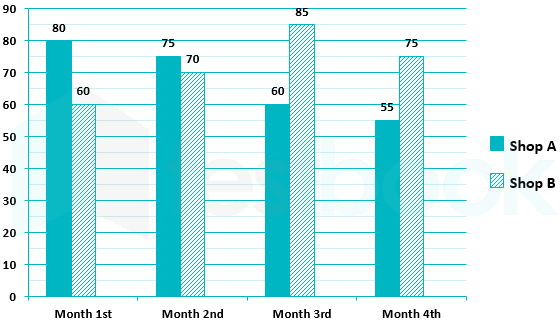Question:
Find the ratio of the number of pens sold by shop A in months 1st and 3rd together to the number of pens sold by shop B in months 2nd and 4th together.
• 27 : 28
• 28 : 29
• 29 : 30
• 26 : 27
• None of these

Number of pen sold by shop A in 1st month = 80

Number of pen sold by shop A in 3rd month = 60

Total number of pen sold by shop A in 1st and 3rd month = 80 + 60 = 140

Number of shop B in 2nd month = 70

Number of shop B in 4th month = 75

Total number of pen sold by shop B in 2nd and 4th month = 70 + 75 = 145

Ratio of Total number of pen sold by shop A in 1st and 3rd month : Total number of pen sold by shop B in 2nd and 4th month = 140 : 145 = 28 : 29

∴ the required ratio = 28 : 29

5. Direction: The given bar graph shows the number of pens sold by two shops A & B in four consecutive months. Study the following data and answer the following questions:Question:
Find the average of the number of pens sold by shop A in 1st and 3rd months and the number of pens sold by shop B in 3rd and 4th month together.
• 75
• 85
• 72
• 78
• None of these

Total numbers of pen sold by shop A in 1st and 3rd month = 80 + 60 = 140

Total numbers of pen sold by shop B in 3rd and 4th month = 85 + 75 = 160

Total numbers of pen sold by shop A in 1st and 3rd month and shop B in 3rd and 4th month = 140 + 160 = 300

Average = 300 / 4 = 75

∴ the required average = 75

6. Direction: The given bar graph shows the number of pens sold by two shops A & B in four consecutive months. Study the following data and answer the following questions:Question:
Find that the number of pens sold by shop A in 1st month is how much percent more than the number of pens sold by shop A in 3rd month.
• 37.5%
• 42.5%
• 33.33%
• 47.5%
• None of these

Number of pens sold by shop A in 1st month = 80

Number of pens sold by shop A in 3rd month = 60

Percent pen sold in month 1 is more than pens sold by shop A in 3rd month = (20/60 )× 100 = 1/3 = 33.33%

7. Direction: The given bar graph shows the number of pens sold by two shops A & B in four consecutive months. Study the following data and answer the following questions:Question:
What is the difference between the number of pens sold by shop A and B together in month 3th and 4th?
• 25
• 15
• 20
• 10
• None of these

Number of pen sold by shop A in 3rd month = 60

Number of pen sold by shop B in 3rd month = 85

Total number of pen sold by shop A and shop B in 3rd month = 60 + 85 = 145

Number of pen sold by shop A in 4th month = 55

Number of pen sold by shop B in 4th month = 75

Total number of pen sold by shop A and shop B in 4th month = 55 + 75 = 130

Difference between number of pen sold A and B in 3rd month and 4th month = 145 – 130 = 15

∴ the required difference = 15

8. Direction: Study the graph carefully and answer the questions given below it.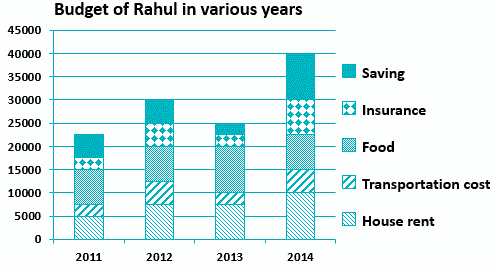Question:
In which year was the percentage increase in insurance maximum?
• In 2013
• In 2102
• In 2014
• In 2011
• In 2014 and in 2013

Given:

Insurance amount increase in 2012: Rs. 2500

Insurance amount increase in 2014: Rs. 5000

Calculation:

In 2014: (5000 / 2500)100

= 200%

In 2012: (2500 / 2500)100

= 100%

9. Direction: Study the graph carefully and answer the questions given below it.Question:
In which year was the change in saving maximum?
• In 2012
• In 2013
• In 2014
• In 2011
• In 2011 and In 2012

Given:

In 2014: Rs. 10,000 – Rs. 2500

= Rs. 7500

10. Direction: Study the graph carefully and answer the questions given below it.Question:
What is the average amount spent on food over the period?
• Rs. 3456
• Rs. 7890
• Rs. 4560
• Rs. 8125
• Rs. 3455

Given:

Amount spent on food in 2011; 2012; 2013 and 2014 is 7500; 7500; 10,000 and 7500

Calculation:

(7500 + 7500 + 10,000 + 7500) / 4 =32500 / 4 = 8125

### AAO Exam-CT 8: Quant (Profit and Loss)